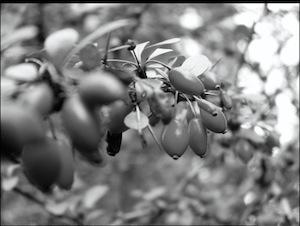Rodolfo Cartas for openphoto.net

### Arrow

An arrow is the term used in category theory as an abstract notion of thing that behaves like a function. In Scalaz, these are `Function1[A, B]`, `PartialFunction[A, B]`, `Kleisli[F[_], A, B]`, and `CoKleisli[F[_], A, B]`. `Arrow` abstracts them all similar to the way other typeclasses abtracts containers.

Here is the typeclass contract for `Arrow`:

``````trait Arrow[=>:[_, _]] extends Category[=>:] { self =>
def id[A]: A =>: A
def arr[A, B](f: A => B): A =>: B
def first[A, B, C](f: (A =>: B)): ((A, C) =>: (B, C))
}
``````

Looks like `Arrow[=>:[_, _]]` extends `Category[=>:]`.

### Category and Compose

``````trait Category[=>:[_, _]] extends Compose[=>:] { self =>
/** The left and right identity over `compose`. */
def id[A]: A =>: A
}
``````

This extends `Compose[=>:]`:

``````trait Compose[=>:[_, _]]  { self =>
def compose[A, B, C](f: B =>: C, g: A =>: B): (A =>: C)
}
``````

`compose` function composes two arrows into one. Using `compose`, `Compose` introduces the following operators:

``````trait ComposeOps[F[_, _],A, B] extends Ops[F[A, B]] {
final def <<<[C](x: F[C, A]): F[C, B] = F.compose(self, x)
final def >>>[C](x: F[B, C]): F[A, C] = F.compose(x, self)
}
``````

The meaning of `>>>` and `<<<` depends on the arrow, but for functions, it’s the same as `andThen` and `compose`:

``````scala> val f = (_:Int) + 1
f: Int => Int = <function1>

scala> val g = (_:Int) * 100
g: Int => Int = <function1>

scala> (f >>> g)(2)
res0: Int = 300

scala> (f <<< g)(2)
res1: Int = 201
``````

### Arrow, again

The type signature of `Arrow[=>:[_, _]]` looks a bit odd, but this is no different than saying `Arrow[M[_, _]]`. The neat things about type constructor that takes two type parameters is that we can write `=>:[A, B]` as `A =>: B`.

`arr` function creates an arrow from a normal function, `id` returns an identity arrow, and `first` creates a new arrow from an existing arrow by expanding the input and output as pairs.

Using the above functions, arrows introduces the following operators:

``````trait ArrowOps[F[_, _],A, B] extends Ops[F[A, B]] {
final def ***[C, D](k: F[C, D]): F[(A, C), (B, D)] = F.splitA(self, k)
final def &&&[C](k: F[A, C]): F[A, (B, C)] = F.combine(self, k)
...
}
``````

`(***)` combines two arrows into a new arrow by running the two arrows on a pair of values (one arrow on the first item of the pair and one arrow on the second item of the pair).

Here’s an example:

``````scala> (f *** g)(1, 2)
res3: (Int, Int) = (2,200)
``````

`(&&&)` combines two arrows into a new arrow by running the two arrows on the same value:

Here’s an example for `&&&`:

``````scala> (f &&& g)(2)
res4: (Int, Int) = (3,200)
``````

Arrows I think can be useful if you need to add some context to functions and pairs.